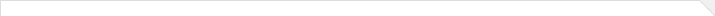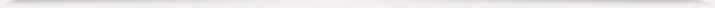报告题目： Cowen-Douglas operators and the third of Halmos' ten problems 报 告 人： 纪奎 教授 报告人所在单位： 河北师范大学 报告日期： 2021-10-22 报告时间： 10:00-11:00 报告地点： 腾讯会议ID: 534 232 190, 密码: 200433 报告摘要： Let T be a bounded linear operator on a complex separable infinite dimensional Hilbert space H. T is called intransitive if it leaves invariant spaces other than 0 or the whole space H; otherwise it is transitive. In 1970, P. R. Halmos raised ten open problems on operator theory. The third problem of Halmos is the following: if an intransitive operator has an inverse, is its inverse also intransitive? In this talk, we introduce some progresses of this problem with the help of Cowen-Douglas operators and spectral analysis.  As the first application, we show that for an invertible hyponormal operator T, if T−1 is intransitive and intσ((T−1)^) is not connected, then T is also intransitive. As the second application, we show that if T−1 has a proper strictly cyclic invariant subspace and there exists a bounded open set Ω which is a connected component of ρ(T−1) such that Ω∩U_0=∅, where U_0 is the connected component of int(σ(T−1)^) containing zero point, then T is intransitive.10-22海报.pdf 本年度学院报告总序号： 246